LSP数据集简介

&& _extended

LSP 地址：

http://sam.johnson.io/research/lsp_dataset.zip

LSP 样本数：2000 个（全身，单人）

LSP_extended 地址：

http://sam.johnson.io/research/lspet_dataset.zip

LSP_extended 样本数：10000 个（全身，单人）

LSP && LSP_extended 共 12000 个标注，节点是以下 14 个：

{1. Right ankle 2. Right knee 3. Right hip 4. Left hip 5. Left knee 6.Left ankle 7.Right wrist 8. Right elbow 9. Right shoulder 10. Left shoulder 11. Left elbow 12. Left wrist 13. Neck 14. Head top}

MPII数据集简介

MPII 人体姿势数据集是人体姿势预估的一个 benchmark，数据集包括了超过 40k 人的 25000 张带标注图片，这些图片是从 YouTube video 中抽取出来的。在测试集中还收录了身体部位遮挡、3D 躯干、头部方向的标注。

MPII 地址：

http://human-pose.mpi-inf.mpg.de/#overview

MPII 样本数：25000 个（单人、多人）

{Head – 0, Neck – 1, Right Shoulder – 2, Right Elbow – 3, Right Wrist – 4, Left Shoulder – 5, Left Elbow – 6, Left Wrist – 7, Right Hip – 8, Right Knee – 9, Right Ankle – 10, Left Hip – 11, Left Knee – 12, Left Ankle – 13, Chest – 14, Background – 15}

MATLAB格式读入

``````
``````
`import scipy.io as sio`

`import numpy as np`

`data = sio.loadmat(self.lsp_anno_path[count])`

`joints = data['joints']`

`joints = np.transpose(joints, (2, 1, 0))`

JSON格式读入

MPII 数据集是以 JSON 格式进行的标注，可以通过 JSON 库进行读入。

``````
``````
`import json`

`anno = json.load(self.mpii_anno_pah)`

``````
``````
`from PIL import Image`

`for idd, joint_idd in enumerate(joints):`

`image_name = "im%s.jpg" % str(idd + 1).zfill(5) if count else "im%s.jpg" % str(idd + 1).zfill(4)`

`joint_id = idd + len(joints) if count else idd`

`im_path = os.path.join(self.lsp_data_path[count], image_name)`

`im = Image.open(im_path)`

`im = np.asarray(im)`

`shape = im.shape`

`bbox = [0, 0, shape, shape]`

`joint_dict[joint_id] = {'imgpath': im_path, 'joints': joint_idd, 'bbox': bbox}`

●  缩放 scale
●  旋转 rotate
●  翻转 flip

``````
``````
`import cv2`

`big_img = cv2.copyMakeBorder(img, add, add, add, add, borderType = cv2.BORDER_CONSTANT, value=self.pixel_means.reshape(-1))`

`#self.show(bimg)`

`bbox = np.array(dic['bbox']).reshape(4, ).astype(np.float32)`

`bbox[:2] += add`

`if 'joints' in dic:`

`process(joints_anno)`

`objcenter = np.array([bbox + bbox / 2., bbox + bbox / 2.])`

`minx, miny, maxx, maxy = compute(extend_border, objcenter, in_size, out_size)`

`img = cv2.resize(big_img[min_y: max_y, min_x: max_x,:], (width, height))`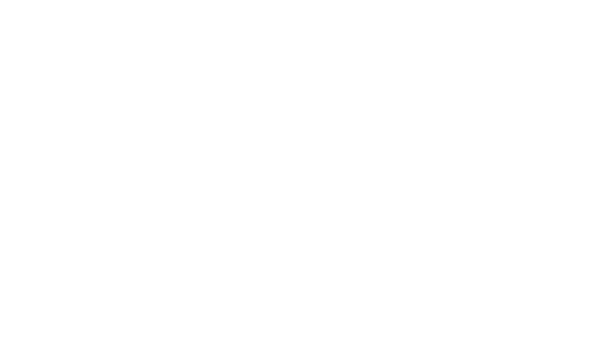▲ 左：原图，右：缩放后

(88.995834, 187.24898)；(107.715065, 160.57408)；(119.648575, 124.30561) (135.3259, 124.53958)；(145.38748, 155.4263)；(133.68799, 165.95587) (118.47862, 109.330215)；(108.41703, 104.65042)；(120.81852, 84.05927) (151.70525, 86.63316)；(162.93677, 101.14057)；(161.29883, 124.773575) (136.0279, 85.93119)；(138.13379, 66.509995)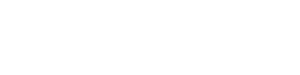``````
``````
`cv2.getRotationMatrix2D((center_x, center_y) , angle, 1.0)`

`newimg = cv2.warpAffine(img, rotMat, (width, height))`

``````
``````
`rot = rotMat[:, : 2]`

`add = np.array([rotMat, rotMat])`

`coor = np.dot(rot, coor) + w`

``````
``````
`def rotate(self, img, cord, anno, center):`

`angle = random.uniform(45, 135)`

`rotMat = cv2.getRotationMatrix2D((center, center) , angle, 1.0)`

`newimg = cv2.warpAffine(img, rotMat, (width, height))`

`for i in range(n):`

`x, y = anno[i], anno[i]`

`coor = np.array([x, y])`

`rot = rotMat[:, : 2]`

`add = np.array([rotMat, rotMat])`

`coor = np.dot(rot, coor) + add`

`label.append((coor, coor))`

`newimg = newimg.transpose(2, 0, 1)`

`train_data[cnt++] = newimg`

`train_label[cnt++] = np.array(label)`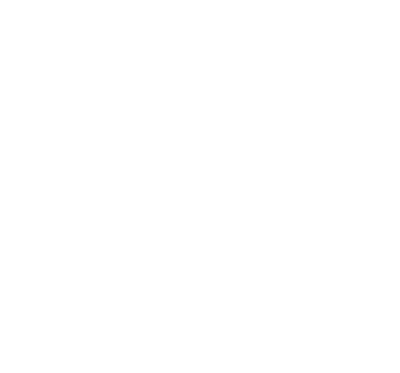``````
``````
`def flip(self, img, cod, anno_valid, symmetry):`

`'''对图片进行翻转'''`

`newimg = cv2.flip(img, 1)`

`train_data[counter] = newimg.transpose(2, 0, 1)`

`'''处理标注点，symmetry是flip后所对应的标注，具体需要自己根据实际情况确定'''`

`for (l, r) in symmetry:`

`cod[l], cod[r] = cod[l], cod[r]`

`for i in range(n):`

`label.append((cod[i],cod[i]))`

`train_label[cnt++] = np.array(label)`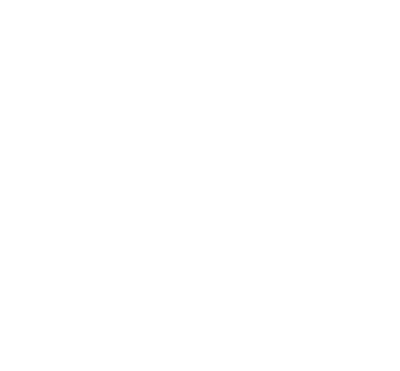``````
``````
`def add_color_noise(self, image, percentage=0.1):`

`noise_img = image`

`'''产生图像大小10%的随机点'''`

`num = int(percentage*image.shape*image.shape)`

`'''添加噪声'''`

`for i in range(num):`

`x = np.random.randint(0,image.shape)`

`y = np.random.randint(0,image.shape)`

`for j in range(3):`

`noise_img[x, y, i] = noise_img[x, y, i] + random.gauss(2,4)`

`noise_img[x, y, i] = 255 if noise_img[x, y, ch] > 255 else 0`

`return noise_img`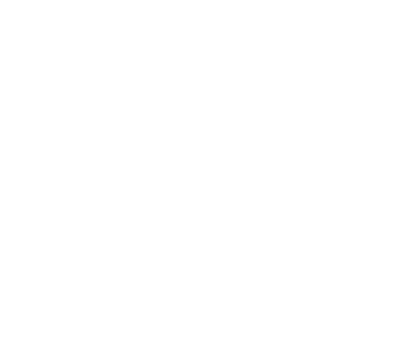1. 从颜色上考虑，还可以做图像亮度、饱和度、对比度变化、PCA Jittering（按照 RGB 三个颜色通道计算均值和标准差后在整个训练集上计算协方差矩阵，进行特征分解，得到特征向量和特征值）；

2. 从图像空间性质上考虑，还可以使用随机裁剪、平移；

3. 从噪声角度，高斯噪声、椒盐噪声、模糊处理；

4. 从类别分布的角度，可以采用 label shuffle、Supervised Data Augmentation（海康威视 ILSVRC 2016 的 report）。

``````
``````
`reader = paddle.batch(self.read_record(test_list, joint_dict,`

`mode = 'train'), batch_size=1)`

`fluid.recordio_writer.convert_reader_to_recordio_file("./work/test_" + str(i) + "_test.recordio",`

`feeder=feeder, reader_creator=reader)`

PCK：检测的关键点与其对应的 groundtruth 之间的归一化距离小于设定阈值的比例。在本篇论文中，作者将图片中心作为身体的位置，并以图片大小作为衡量身体尺寸的标准。

[email protected] on LSP && LSP-extended：以驱干直径为归一化标准。

[email protected] on MPII：以头部为归一化标准。